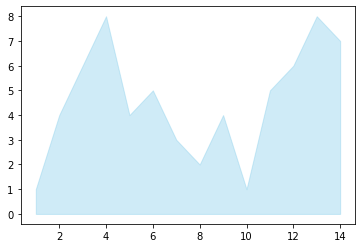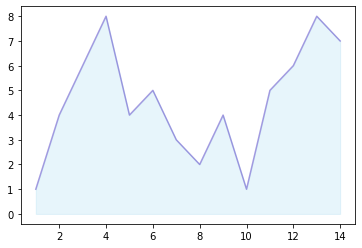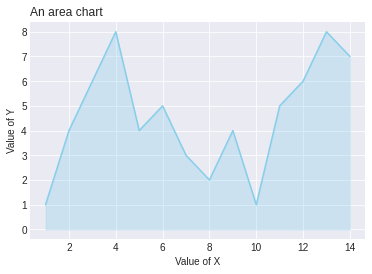# Improved Area Chart

The previous post describes how to make a basic area chart. This page aims to describe a few customisation you can apply to your area chart.

It is highly advised to change the default color of matplotlib. Moreover, it is quite common to use a color with transparency, and add a line with a stronger colour on the top of the area.

You can pass the `color` parameter to change the color of the area and the `alpha` parameter to change the color transparancy of the area to the `fill_between()` function. You can also add a line with the `plot()` function and change its color to make the edge of the area marked.

``````# libraries
import numpy as np
import matplotlib.pyplot as plt

# create data
x=range(1,15)
y=[1,4,6,8,4,5,3,2,4,1,5,6,8,7]

# Change the color and its transparency
plt.fill_between( x, y, color="skyblue", alpha=0.4)

# Show the graph
plt.show()

# Same, but add a stronger line on top (edge)
plt.fill_between( x, y, color="skyblue", alpha=0.2)
plt.plot(x, y, color="Slateblue", alpha=0.6)
# See the line plot function to learn how to customize the plt.plot function

# Show the graph
plt.show()``````Last but not least, it is often worth it to change the style before doing your chart in order to plot a nice looking graph. Moreover, don’t forget to add a title and give names to the axis:

``````# libraries
import numpy as np
import matplotlib.pyplot as plt

# create data
x=range(1,15)
y=[1,4,6,8,4,5,3,2,4,1,5,6,8,7]

# Change the style of plot
plt.style.use('seaborn-darkgrid')

# Make the same graph
plt.fill_between( x, y, color="skyblue", alpha=0.3)
plt.plot(x, y, color="skyblue")👋 This document is a work by Yan Holtz. Any feedback is highly encouraged. You can fill an issue on Github, drop me a message onTwitter, or send an email pasting `yan.holtz.data` with `gmail.com`.